Next: Reference priors Up: General principle based priors Previous: Transformation invariance

### Maximum-entropy priors

Another principle-based approach to assigning priors is based on in the Maximum Entropy principle (Jaynes 1957a, also 1983, 1998, Tribus 1969, von der Linden 1995, Sivia 1997, and Fröhner 2000). The basic idea is to choose the prior function that maximizes the Shannon-Jaynes information entropy,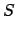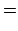(105)

subject to whatever is assumed to be known about the distribution. The largeris, the greater is our ignorance about the uncertain value of interest. The value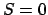is obtained for a distribution that concentrates all the probability into a single value. In the case of no constraint other than normalization, (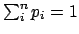),is maximized by the uniform distribution,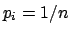, which is easily proved using Lagrange multipliers. For example, if the variable is an integer between 0 and 10, a uniform distribution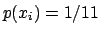gives. Any binomial distribution with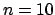gives a smaller value, with a maximum of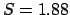for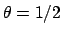and a limit offor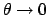or or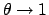, whereis now the parameter of the binomial that gives the probability of success at each trial.

Two famous cases of maximum-entropy priors for continuous variables are when the only information about the distribution is either the expected value or the expected value and the variance. Indeed, these are special cases of general constraints on the moments of the distribution(see Tab. 1). For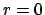and 1,is equal to unity and to the expected value, respectively. First and second moment together provide the variance (see Tab. 1 and Sect. 5.6). Let us sum up what the assumed knowledge on the various moments provides [see e.g. (Sivia 1997, Dose 2002)].

Knowledge aboutNormalization alone provides a uniform distribution over the interval in which the variable is defined: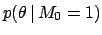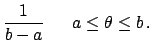(106)

This is the extension to continuous variables of the discrete case we saw above.
Knowledge aboutand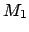[i.e. about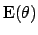]

Adding to the constraintthe knowledge about the expectation of the variable, plus the requirement that all non-negative values are allowed, an exponential distribution is obtained:(107)

Knowledge about,and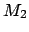[i.e. aboutand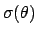]

Finally, the further constraint provided by the standard deviation (related to first and second moment by the equation) yields a prior with a Gaussian shape independently of the range of, i.e.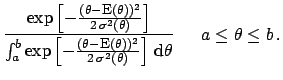The standard Gaussian is recovered whenis allowed to be any real number.
Note, however, that the counterpart of Eq. (105) for continuous variables is not trivial, since allof Eq. (105) tend to zero. Hence the analogous functional form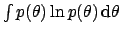no longer has a sensible interpretation in terms of uncertainty, as remarked by Bernardo and Smith (1994). The Jaynes' solution is to introduce a `reference' density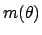to make entropy invariant under coordinate transformation via. (It is important to remark that the first and the third case discussed above are valid under the assumption of a unity reference density.) This solution is not universally accepted (see Bernardo and Smith 1994), even though it conforms to the requirements of dimensional analysis. Anyhow, besides formal aspects and the undeniable aid of Maximum Entropy methods in complicate problems such as image reconstruction (Buck and Macauly 1991), we find it very difficult, if not impossible at all, that a practitioner holds that status of knowledge which give rise to the two celebrated cases discussed above. We find more reasonable the approach described in Sect. 8.2, that goes the other way around: we have a rough idea of where the quantity of interest could be, then we try to model it and to summarize it in terms of expected value and standard deviation. In particular, we find untenable the position of those who state that Gaussian distribution can only be justified by Maximum Entropy principle.Next: Reference priors Up: General principle based priors Previous: Transformation invariance
Giulio D'Agostini 2003-05-13